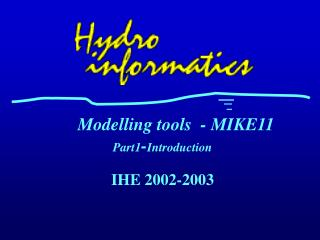# Modelling tools - MIKE11 Part1 - Introduction - PowerPoint PPT PresentationDownload PresentationModelling tools - MIKE11 Part1 - Introduction

Presentation Description
Download Presentation## Modelling tools - MIKE11 Part1 - Introduction

- - - - - - - - - - - - - - - - - - - - - - - - - - - E N D - - - - - - - - - - - - - - - - - - - - - - - - - - -
##### Presentation Transcript

1. Modelling tools - MIKE11Part1-Introduction

2. What is a model ? • ‘A model is a caricature of reality.´ R. May • A model is a simplification of reality that retains enough aspects of the original system to make it useful to the modeler • Models may take many forms • phisical models ( hydrologic models of watersheds; scales models of ships) • conceptual (differential equations, optimization) • simulation models

3. The modelling process • Understand the problem • reason to model a system ( e.g. what if a dam is built?) • collect and analyse data • Choosing variables • Set up mathematical model • describe situation • write mathematical explanation using variables • Assumptions about the system • Construction of the mathematical model • Computer simulation • computer program • input data and runs • validation • Simulation experiments • interpret the solution, test outcomes • improve the model

4. Classification of modelling packages • According to what is computed • water surface profiles (HEC2) • flood waves (DAMBRK) • water quality in rivers (QUAL2E) • habitat modelling (PHABSIM) • How many dimensions are used • 1D models (MIKE11, SOBEK) • 2D models • 3D models (DELFT3D) • Particulars of the numerical methods • finite differences • finite elements • boundary elements • etc

5. MIKE11 - General description • Software package developed by Danish Hydraulic Institute (DHI) for simulation of flow, sediment transport and water quality in estuaries, river, irrigation system and similar water bodies • User - friendly tool for design, management and operation of river basins and channel networks

6. Implementation • Mike 11 includes the following modules • HD - hydrodynamic - simulation of unsteady flow in a network of open channels. Result is time series of discharges and water levels; • AD - advection dispersion • WQ - water quality

7. Theory • Open channel flow- Saint Venant equations (1D) • continuity equation (mass conservation) • momentum equation (fluid momentum conservation) • Assumptions • water is incompresible and homogeneous • bottom slope is small • flow everywhere is paralel to the bottom ( i.e. wave lengths are large compared with water depths)

8. Hydraulic variables

9. Equations • Mass conservation • Momentum conservation

10. Equations variables • Independent variables • space x • time t • Dependent variables • discharge Q • water level h • All other variables are function of the independent or dependent variables

11. Cross section i-1 Cross section i Cross section i+1 Time Time step j+1 Reach Time step j Time step j-1 Space Flow description • Depending on how many terms are used in momentum equations • full Saint Venant equations (dynamic wave) • explicit methods • implicit methods

12. Flow description • Neglect first two terms • Diffusive wave ( backwater analysis) • Neglect three terms • Kinematic wave (relatively steep rivers without backwater effects)

13. i-1 i i+1 Time Time step n+1 Q h7 6 Time step n+1/2 Q h5 Q 4 h3 h1 2 Time step n Center point Space Solution scheme • Equations are transformed to a set of implicit finite difference equations over a computational grid • alternating Q - and H points, where Q and H are computed at each time step • numerical scheme - 6 point Abbott-Ionescu scheme

14. Solution scheme • Boundary conditions • external boundary conditions - upstream and downstream; • internal “boundary conditions” - hydraulic structures ( here Saint Venant equation are not applicable) • Initial condition • time t=0

15. Choice of boundary conditions • Typical upstream boundary conditions • constant discharge from a reservoir • a discharge hydrograph of a specific event • Typical downstream boundary conditions • constant water level • time series of water level ( tidal cycle) • a reliable rating curve ( only to be used with downstream boundaries)

16. Discretization - branches

17. Discretization - branches

18. Discretization - cross sections • Required at representative locations throughout the branches of the river • Must accurately represent the flow changes, bed slope, shape, flow resistance characteristics

19. Discretization - cross section • Friction formulas • Chezy • Manning • For each section a curve is made with wetted area, conveyance factor, hydraulic radius as a function of water level R h

20. Avoiding Errors • Hydraulic jump can not be modelled, but upstream and downstream condition can • Stability conditions • topographic resolution must be sufficiently fine (x) • time step • should be fine enough to provide accurate representation of a wave • if structure are used smaller time step is required • use Courant condition to determine time step • or velocity condition

21. Structures • Broadcrested weirs • Special weirs • User-defined culverts • Q-h calculated culverts • Dam break structure